GeeksforGeeks App
Open AppBrowser
Continue

# How to export Pandas DataFrame to a CSV file?

Let us see how to export a Pandas DataFrame to a CSV file. We will be using the `to_csv()` function to save a DataFrame as a CSV file.

## DataFrame.to_csv()

Syntax : to_csv(parameters)
Parameters :

• path_or_buf : File path or object, if None is provided the result is returned as a string.
• sep : String of length 1. Field delimiter for the output file.
• na_rep : Missing data representation.
• float_format : Format string for floating point numbers.
• columns : Columns to write.
• header : If a list of strings is given it is assumed to be aliases for the column names.
• index : Write row names (index).
• index_label : Column label for index column(s) if desired. If None is given, and header and index are True, then the index names are used.
• mode : Python write mode, default ‘w’.
• encoding : A string representing the encoding to use in the output file.
• compression : Compression mode among the following possible values: {‘infer’, ‘gzip’, ‘bz2’, ‘zip’, ‘xz’, None}.
• quoting : Defaults to csv.QUOTE_MINIMAL.
• quotechar : String of length 1. Character used to quote fields.
• line_terminator : The newline character or character sequence to use in the output file.
• chunksize : Rows to write at a time.
• date_format : Format string for datetime objects.
• doublequote : Control quoting of quotechar inside a field.
• escapechar : String of length 1. Character used to escape sep and quotechar when appropriate.
• decimal : Character recognized as decimal separator. E.g. use ‘,’ for European data.

Returns : None or str

Example 1 :

 `# importing the module``import` `pandas as pd`` ` `# creating the DataFrame``my_df ``=` `{``'Name'``: [``'Rutuja'``, ``'Anuja'``], ``         ``'ID'``: [``1``, ``2``], ``         ``'Age'``: [``20``, ``19``]}``df ``=` `pd.DataFrame(my_df)`` ` `# displaying the DataFrame``print``(``'DataFrame:\n'``, df)``  ` `# saving the DataFrame as a CSV file``gfg_csv_data ``=` `df.to_csv(``'GfG.csv'``, index ``=` `True``)``print``(``'\nCSV String:\n'``, gfg_csv_data)`

Output :
Before executing the code: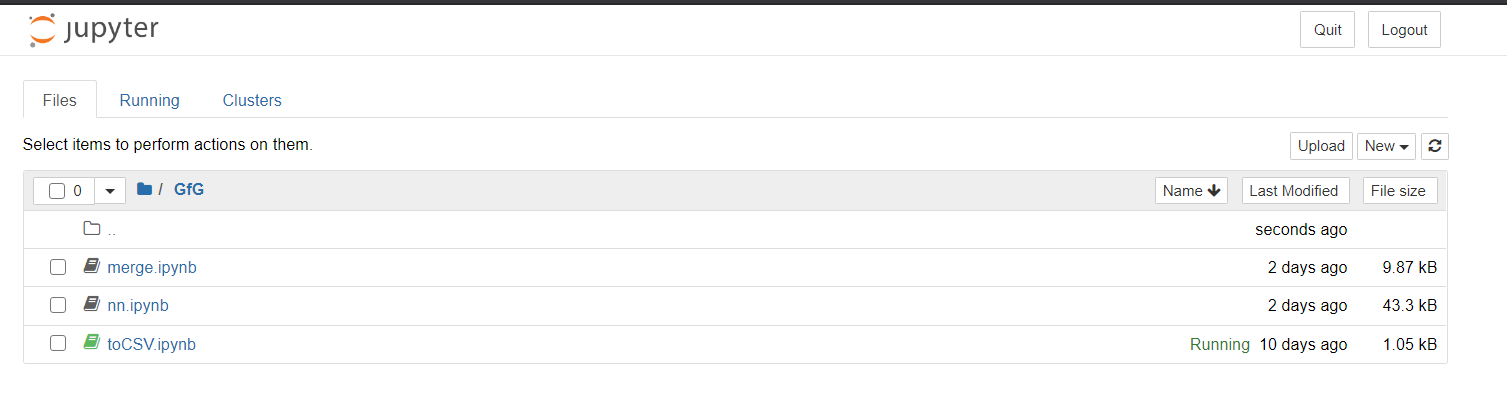After executing the code: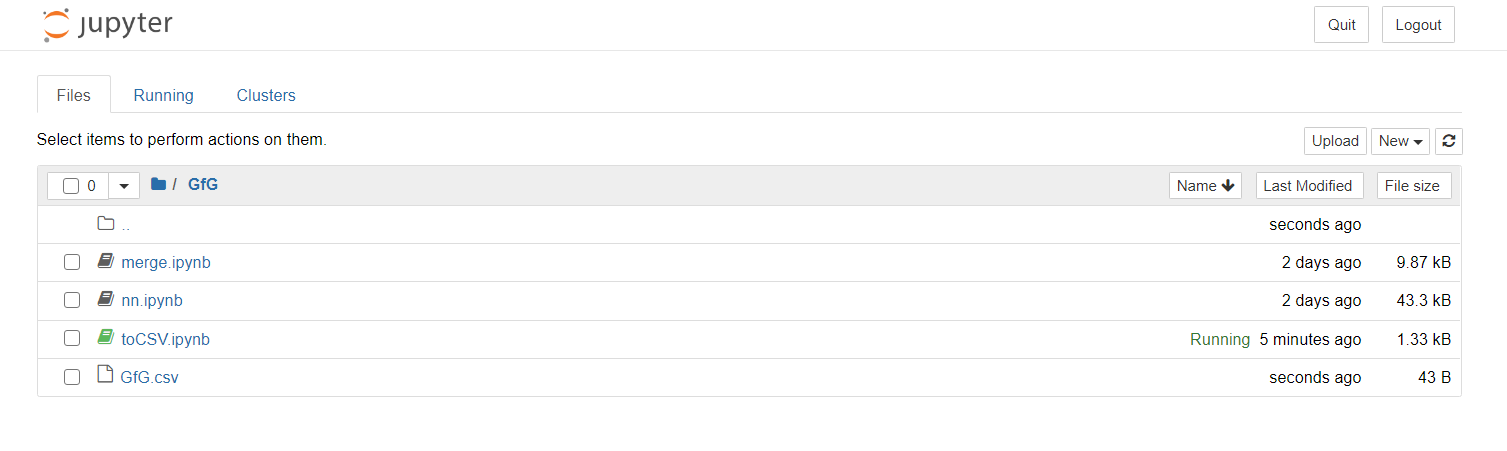We can clearly see the .csv file created.

Also, the output of the above code includes the index, as follows.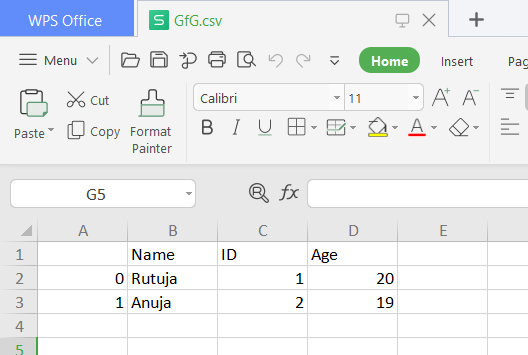Example 2 : Converting to a CSV file without the index. If we wish not to include the index, then in the `index `parameter assign the value `False`.

 `# importing the module``import` `pandas as pd`` ` `# creating the DataFrame``my_df ``=` `{``'Name'``: [``'Rutuja'``, ``'Anuja'``], ``         ``'ID'``: [``1``, ``2``], ``         ``'Age'``: [``20``, ``19``]}``df ``=` `pd.DataFrame(my_df)`` ` `# displaying the DataFrame``print``(``'DataFrame:\n'``, df)``  ` `# saving the DataFrame as a CSV file``gfg_csv_data ``=` `df.to_csv(``'GfG.csv'``, index ``=` `False``)``print``(``'\nCSV String:\n'``, gfg_csv_data)`

Output: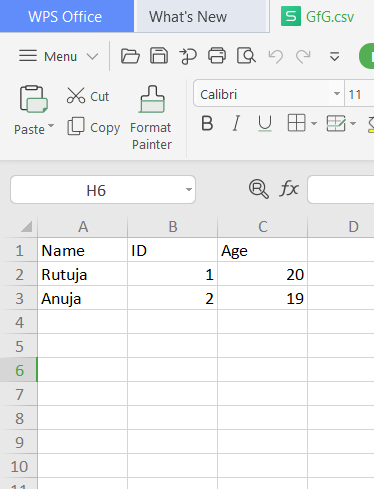Example 3 : Converting to a CSV file without the header of the rows. If we wish not to include the header, then in the `header`parameter assign the value `False`.

 `# importing the module``import` `pandas as pd`` ` `# creating the DataFrame``my_df ``=` `{``'Name'``: [``'Rutuja'``, ``'Anuja'``], ``         ``'ID'``: [``1``, ``2``], ``         ``'Age'``: [``20``, ``19``]}``df ``=` `pd.DataFrame(my_df)`` ` `# displaying the DataFrame``print``(``'DataFrame:\n'``, df)``  ` `# saving the DataFrame as a CSV file``gfg_csv_data ``=` `df.to_csv(``'GfG.csv'``, header ``=` `False``)``print``(``'\nCSV String:\n'``, gfg_csv_data)`

Output: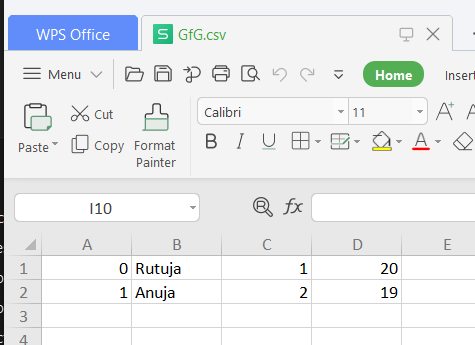My Personal Notes arrow_drop_up